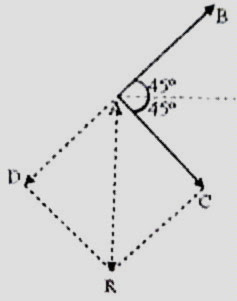## Pages

`“Life is like riding a bicycle.  To keep your balance you must keep moving.”–Albert Einstein`

## Wednesday, August 27, 2008

### Kinematics for AP Physics B and C – Multiple Choice Questions on Two Dimensional Motion

We will discuss free response questions on kinematics after discussing multiple choice questions on two dimensional motion. Here are some typical MCQ’s for practice:

(1) A particle A of mass 4m is released from rest from a point P at the top edge of a tower. Simultaneously, particles B and C of masses m and 2m respectively are projected horizontally with equal velocities. Which one of the following statements is correct? (Neglect air resistance)

(a) Particle A will reach the ground first

(b) Particles B and C will not reach the ground simultaneously

(c) Particle B will reach the ground last

(d) Statements (a), (b) and (c) are correct

(e) All the above statements are incorrect

The vertical acceleration of the particles is the same and is the gravitational acceleration g. The initial vertical component of velocity is zero for all the particles. Since the vertical displacement is the same, all the particles will reach the ground simultaneously. So the correct option is (e).

[Gravitational acceleration is independent of the mass of the particle and you should not be distracted].

(2) A particle of mass m is projected from the ground level with a velocity v making an angle 60º with the horizontal. What will be the angular momentum of the particle about the point of projection just before it hits the ground? (Neglect air resistance)(a) 3mv3/4g

(b) 3mv3/2g

(c) mv3/2g

(d) 3mv3/4g

(e) √3 mv3/2g

The velocity of the particle when it returns to the ground level will have magnitude v and will be inclined at 60º with the horizontal as shown. Therefore, its angular momentum with respect to the point of projection A will be mv×AC where AC is drawn perpendicular to the direction (CB) of the projectile just before it hits the ground.

But AC = AB sin 60º = [(v2sin120º)/g]×sin60º

= (v2/g)(√3/2)×(√3/2) = 3v2/4g

[Note that the horizontal range (R) of a projectile is given by R = (v02sin 2θ0)/g]

Therefore, angular momentum = mv×3v2/4g = 3mv3/4g

(3) The magnitude of the velocity of a projectile at the maximum height is half the magnitude of its initial velocity. If the initial velocity is u, the maximum height reached is

(a) 3u2/2g

(b) 3u2/4g

(c) 3u2/8g

(d) √3u2/2g

(e) √3u2/4g

At maximum height the projectile has horizontal velocity only . Its value is ucosθ0 throughout where θ0 is the angle of projection. Therefore we have ucosθ0 = u/2 so that θ0 = 60º

Maximum height reached, H = (u2sin2θ0)/2g = (u2sin260º)/2g = 3 u2/8g

(4) A stone is projected at an angle θ with the horizontal. If the initial kinetic energy of the stone is E, what is its kinetic energy at the topmost point of its trajectory? (Neglect air resistance).

(a) Zero

(b) E cosθ

(c) E/2

(d) E cos2θ

(e) E sin2θ

We have E = ½ mv02 where v0 is the velocity of projection of the stone (of mass m).

Since at the maximum height the projectile has horizontal velocity only and its value is v0cosθ, the kinetic energy at the maximum height is ½ mv02cos2θ = E cos2θ.

(5) A bullet of mass m is fired with velocity v from an air gun at an angle of 45º to the horizontal. The magnitude of the change in momentum of the bullet on arriving at the horizontal plane passing through the point of firing is

(a) zero

(b) mv

(c) 2mv

(d) mv/√2

(e) √2 mvThe initial momentum of magnitude mv of the bullet is inclined at 45º with the horizontal and is represented by the vector AB in the adjoining figure. The momentum when the bullet reaches the horizontal plane passing through the point of projection during its return to the ground is represented by the vector AC. Its magnitude is mv itself and it is inclined at 45º with the horizontal. The change in the momentum is vector AC – vector AB. The vector –AB is represented by the vector AD so that vector (AC – AB) = vector AR. Since the magnitudes of the vectors AC and AB are the same and equal to mv and the angle between them is 90º, the magnitude of the change in momentum of the bullet is √(m2v2 + m2v2) = √2 mv.

The figure showing the vectors is given here just to make things clear. You should be able to find the answer without using the figure.

You can arrive at the answer much more easily if you remember that there is no change in the horizontal component of momentum. The vertical component of momentum which has magnitude mv sin45º gets reversed so that the change in momentum is mv sin45º (mv sin 45º) = 2 mv sin 45º = √2 mv.

In the next post we will discuss some more questions from this section.

You will find a useful post on AP Physics Kinematics here